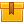# Problem Solving Techniques (A8507)

## Prerequisites

This lesson is designed for participants familiar with the principles of statistical process charts. Basic mathematical skills and graphing techniques are also required.

## Description

This lesson covers several problem-solving techniques including brainstorming, Pareto diagrams, cause and effect diagrams, and scatter diagrams. The lesson focuses on how to collect data and display it graphically as well as how to apply graphic tools to problem solving.

## Objectives

Construct a Pareto diagram from data provided by tally charts, plant records, and control charts. Define the Pareto principle of 80/20 rule. Calculate the cumulative frequencies and percentages of defect categories and plot them on a Pareto diagram. Construct a cause and effect diagram. Extend a fishbone diagram through logical backward analysis of a cause. Distinguish between dependent and independent variables. Construct a scatter diagram as well as interpret it for relationships between variables.

## ContentThis lesson covers several problem-solving techniques including brainstorming, Pareto diagrams, cause and effect diagrams, and scatter diagrams. The lesson focuses on how to collect data and display it graphically as well as how to apply graphic tools to problem solving.

•Problem Solving Techniques (A8507)

0
0 Reviews